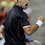# Are you good at $Balancing$?

Here's a problem I've been trying for ages.... $C_{2}H_{5}OH+ I_{2}+ OH^{-}\longrightarrow\ CHI_{3}+HCOO^{-}+H_{2}O$

$Question:$ Find the stoichiometric coefficients of each of the given compounds involved in the reaction by balancing it( preferably by oxidation no.or Ion-electron method).

Do try this question and post a nice solution for it!Note by Samarpit Swain
5 years, 9 months ago

This discussion board is a place to discuss our Daily Challenges and the math and science related to those challenges. Explanations are more than just a solution — they should explain the steps and thinking strategies that you used to obtain the solution. Comments should further the discussion of math and science.

When posting on Brilliant:

• Use the emojis to react to an explanation, whether you're congratulating a job well done , or just really confused .
• Ask specific questions about the challenge or the steps in somebody's explanation. Well-posed questions can add a lot to the discussion, but posting "I don't understand!" doesn't help anyone.
• Try to contribute something new to the discussion, whether it is an extension, generalization or other idea related to the challenge.

MarkdownAppears as
*italics* or _italics_ italics
**bold** or __bold__ bold
- bulleted- list
• bulleted
• list
1. numbered2. list
1. numbered
2. list
Note: you must add a full line of space before and after lists for them to show up correctly
paragraph 1paragraph 2

paragraph 1

paragraph 2

[example link](https://brilliant.org)example link
> This is a quote
This is a quote
    # I indented these lines
# 4 spaces, and now they show
# up as a code block.

print "hello world"
# I indented these lines
# 4 spaces, and now they show
# up as a code block.

print "hello world"
MathAppears as
Remember to wrap math in $$ ... $$ or $ ... $ to ensure proper formatting.
2 \times 3 $2 \times 3$
2^{34} $2^{34}$
a_{i-1} $a_{i-1}$
\frac{2}{3} $\frac{2}{3}$
\sqrt{2} $\sqrt{2}$
\sum_{i=1}^3 $\sum_{i=1}^3$
\sin \theta $\sin \theta$
\boxed{123} $\boxed{123}$

Sort by:

Sorry for the way too late response dude !!!

While balancing ignore $H_2 O$ and $OH^{-}$ keeping in mind that the medium is basic .(Basically we don't need them as such since they are bound to come in the scene at the end ) . Now, balance the rest i.e. $C_{2}H_{5}OH+ I_{2} \longrightarrow CHI_{3}+HCOO^{-}$ , you will get $2C_{2}H_{5}OH+ 3I_{2}+ 12OH^{-}\longrightarrow\ 2CHI_{3}+2HCOO^{-}+10H_{2}O$ but here you'll notice that the charge is not balanced on the RHS so I think the correct answer is $2C_{2}H_{5}OH+ 3I_{2}+ 12OH^{-}\longrightarrow\ 2CHI_{3}+2HCOO^{-}+10H_{2}O + 10e^{-}$

- 5 years, 9 months ago

I'm really, really sorry for the late response.

I think your method is absolutely fine, but are you sure that you can have excess electrons at the end of such reactions? I've never come across such an equation before.

Also the law of conservation of mass is violated(though negligibly), since most of the theoretical reactions give an ideal perspective.

- 5 years, 8 months ago

Well , I don't think we will be able to manage without the extra electrons . Do you have anything else in mind ?

@Ronak Agarwal , @Pranjal Jain , @Deepanshu Gupta : What would you guys have done ?

- 5 years, 8 months ago

I believe mechanism of iodoform reaction is the key.

- 5 years, 8 months ago

Can you explain it a bit more , please?

- 5 years, 8 months ago

See this. Replace $-R$ by $-H$ and the disproportionation of $I_2$ will be a bit different.

$3I_2+6OH^-\rightarrow 5I^-+IO_3^-+3H_2O$

Just add all elementary reactions to get stoichiometric coefficients.

- 5 years, 8 months ago

Thanks

- 5 years, 8 months ago

Have you tried taking $C_2H_5OH + I_2 \rightarrow CHI_3$ and $C_2H_5OH \rightarrow HCOO^-$ as the two half reactions and solving? Not going to post a full solution because exams.

- 5 years, 9 months ago

Yup, I've tried that way, didn't help:(

- 5 years, 9 months ago